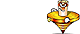Unit Conversion Software Web Widgets Loan Calculator Currency Rates Country Flags Unit Converter
 Faq Help# Torque ConverterContact
 From: To: dyne centimeter [dyn*cm]dyne meter [dyn*m]dyne millimeter [dyn*mm]gram-force centimeter [gf*cm]gram-force meter [gf*m]gram-force millimeter [gf*mm]kilogram-force centimeter [kgf*cm]kilogram-force meter [kgf*m]kilogram-force millimeter [kgf*mm]kilonewton meter [kN*m]newton centimeter [N*cm]newton meter [N*m]newton millimeter [N*mm]ounce-force foot [ozf*ft]ounce-force inch [ozf*in]pound-force foot [lbf*ft]pound-force inch [lbf*in] dyne centimeter [dyn*cm]dyne meter [dyn*m]dyne millimeter [dyn*mm]gram-force centimeter [gf*cm]gram-force meter [gf*m]gram-force millimeter [gf*mm]kilogram-force centimeter [kgf*cm]kilogram-force meter [kgf*m]kilogram-force millimeter [kgf*mm]kilonewton meter [kN*m]newton centimeter [N*cm]newton meter [N*m]newton millimeter [N*mm]ounce-force foot [ozf*ft]ounce-force inch [ozf*in]pound-force foot [lbf*ft]pound-force inch [lbf*in] Result:How to use Torque Converter
Select the unit to convert from in the input units list. Select the unit to convert to in the output units list. Enter the value to convert from into the input box on the left. The conversion result will immediately appear in the output box.Download Torque Unit Converter
our powerful software utility that helps you make easy conversion between more than 2,100 various units of measure in more than 70 categories. Discover a universal assistant for all of your unit conversion needs - download the free demo version right away!Copyright © 1998-2009 UnitConversion.org Privacy & Terms | About | Faq | Help | Contact | Link to Us